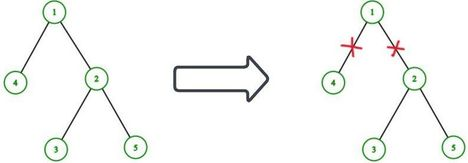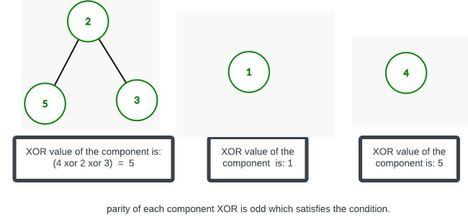# Same Parity SplitAhasan_1999CUET CSE Fest 2022 - Inte...
Limits 2s, 512 MB

A tree is a connected graph without a cycle. Given an undirected tree with $n$ nodes labeled from $1$ to $n$. Each node has a certain weight assigned to it given by an integer array $A$ of size $n$.

Eren wants to split the tree into several different connected components by deleting $K$ different edges from the tree. Now, he is wondering what is the minimum value of $K$ such that after deleting $K$ different edges, the XOR value of each resulting component has the same parity.

In other words, deleting $K$ different edges will split the tree into $K+1$ different connected components. Now, let’s say the XOR of each connected component is $X_i$ for all $(1 \leq i \leq K+1)$. Either all $X_i$ must be even or all $X_i$ must be odd. The value of $K$ must be at least $1$.

XOR of a connected component is defined by the bitwise XOR of the weight of all the nodes in that component.

Help Eren to solve the problem or print $-1$ if it is not possible.

## Input

Each test contains multiple test cases. The first line contains a single integer $T$ $(1 ≤ T ≤ 4 \times 10^{4})$— the number of test cases. Description of the test cases follows.

The first line of each test case contains a single integer $n$ $(2 ≤ n≤ 5 \times 10^{5})$ — the number of vertices in the tree.

The second line contains $n$ space-separated integers $A_1,A_2,…,A_n(1≤ A_i ≤ 10^9)$ — the weight of the vertices.

Next $n−1$ lines contain the edges of the tree — one per line. Each line contains two integers $u$ and $v$ $(1 ≤ u,v ≤n; u ≠ v)$ representing one edge of the tree. It's guaranteed that the given edges form a tree.

It is guaranteed that the sum of $n$ over all test cases does not exceed $5 \times 10^{5}$

## Output

For each test case, first, print $-1$ if it is not possible to split, otherwise, print the minimum value of $K$.

If it is possible to split, print an additional line containing $K$ space-separated integers $P_1, P_2, …, P_K$ $( 1 \leq P_i \leq n - 1)$— the index of the deleted edge. You can print the index in any order.

If there are multiple possible answers, print any of them.

## Sample

InputOutput
2
5
1 4 2 5 3
1 2
1 4
3 2
2 5
3
2 1 4
1 2
2 3

2
1 2
-1


In the first case, one of the possible solutions is to delete the first two edges. So, the minimum value of K is 2.In the second case, it is not possible to split the tree satisfying the condition.

### Submit